# Integer facts for kids

Kids Encyclopedia Facts
(Redirected from Integers)

In mathematics, integers are the natural numbers and their negatives. Integers can also be shown on a number line as follows: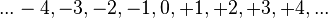${...-4, -3, -2, -1, 0, +1, +2, +3, +4,...}\,\!$

In particular, zero is also an integer, but it is not positive nor negative. "Integer" is another word for "whole". An integer is a rational number with no "fraction", or part. An integer is a decimal number with all zeros after the decimal separator. For example, the integer 17 is the same as the decimal 17.0 or 17.0000.

An integer has a next smaller number and a next larger number. There is no smallest integer, just as there is no largest integer. Each integer is either larger than, equal to, or smaller than any other integer. Consecutive integers are integers that come after each other (as in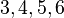${3, 4, 5, 6}$).

The sum of integers is an integer. The difference between integers is an integer. The product of integers is an integer (for example, (12 + 2345 x (67 - 8) ) x 9 is an integer). An integer divided by an integer is sometimes not an integer (as in the case of 123 / 45).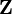$\mathbf{Z}$ or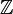$\mathbb{Z}$ is the name of the set of integers, and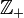$\mathbb{Z}_+\!$ the set of positive integers. ($\mathbf{Z}$, +, 0) is an abelian group.

The number of integers is infinite. The cardinal number of$\mathbf{Z}$ is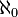$\aleph_0$. The ordinal number of$\mathbf{Z}$ is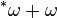$^*\omega + \omega$.

To sum up, an integer is a whole number that has no decimals.

## In programming

In some programming languages, like C, there are types called "int" or "integer."Integer Facts for Kids. Kiddle Encyclopedia.